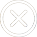400-1888-810

# 东方财富rsi选股公式是什么？终于有人说透了！

248次　 2023-05-17　分类：证券文章

DIFF:=EMA(CLOSE,12) - EMA(CLOSE,26);
DEA:=EMA(DIFF,9);
MACD:=2*(DIFF-DEA);
A1:=BARSLAST(REF(CROSS(DIFF,DEA),1));
B1:=REF(C,A1+1)>C AND REF(DIFF,A1+1)
A2:=BARSLAST(REF(CROSS("KDJ.K"(9,3,3),"KDJ.D"(9,3,3)),1));
B2:=REF(C,A2+1)>C AND REF("KDJ.K"(9,3,3),A2+1)<"KDJ.K"(9,3,3) AND CROSS("KDJ.K"(9,3,3),"KDJ.D"(9,3,3));
A3:=BARSLAST(REF(CROSS("RSI.RSI1"(6,12,24),"RSI.RSI2"(6,12,24)),1));
B3:=REF(C,A3+1)>C AND REF("RSI.RSI1"(6,12,24),A3+1)<"RSI.RSI1"(6,12,24) AND CROSS("RSI.RSI1"(6,12,24),"RSI.RSI2"(6,12,24));
C1:=BARSLAST(REF(CROSS(DEA,DIFF),1));
D1:=REF(C,C1+1)DIFF AND CROSS(DEA,DIFF);
C2:=BARSLAST(REF(CROSS("KDJ.D"(9,3,3),"KDJ.K"(9,3,3)),1));
D2:=REF(C,C2+1)"KDJ.K"(9,3,3) AND CROSS("KDJ.D"(9,3,3),"KDJ.K"(9,3,3));
C3:=BARSLAST(REF(CROSS("RSI.RSI2"(6,12,24),"RSI.RSI1"(6,12,24)),1));
D3:=REF(C,C3+1)"RSI.RSI1"(6,12,24) AND CROSS("RSI.RSI2"(6,12,24),"RSI.RSI1"(6,12,24));
RSI底背:B3>0;

1、如下图所示，用到的功能为东方财富的“条件选股”。短期RSI指标上穿长期RSI指标的情况被广泛知晓，就会出现RSI指标金叉的情况，大概率是在传达买入信号，所以我们可以利用RSI指标这个特征进行选股。

2、如下图所示，RSI指标被用来指示市场的超买和超卖状况，当RSI指标跌破20的数值时，表示股票当前处在超卖状态，股价反弹上升的几率比较高。## 证券问题标签

dr股票

xd是什么意思

st股票

KDJ